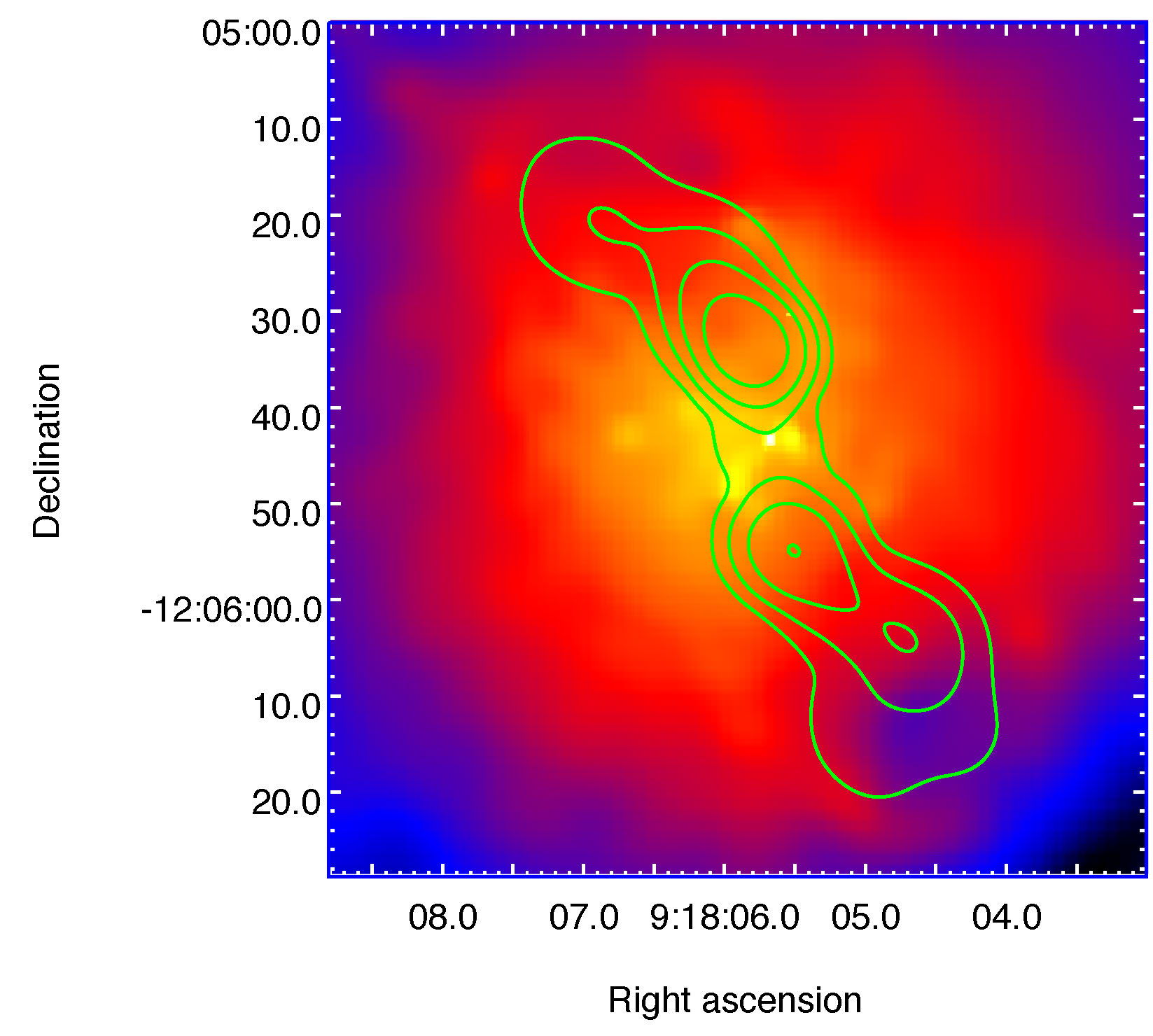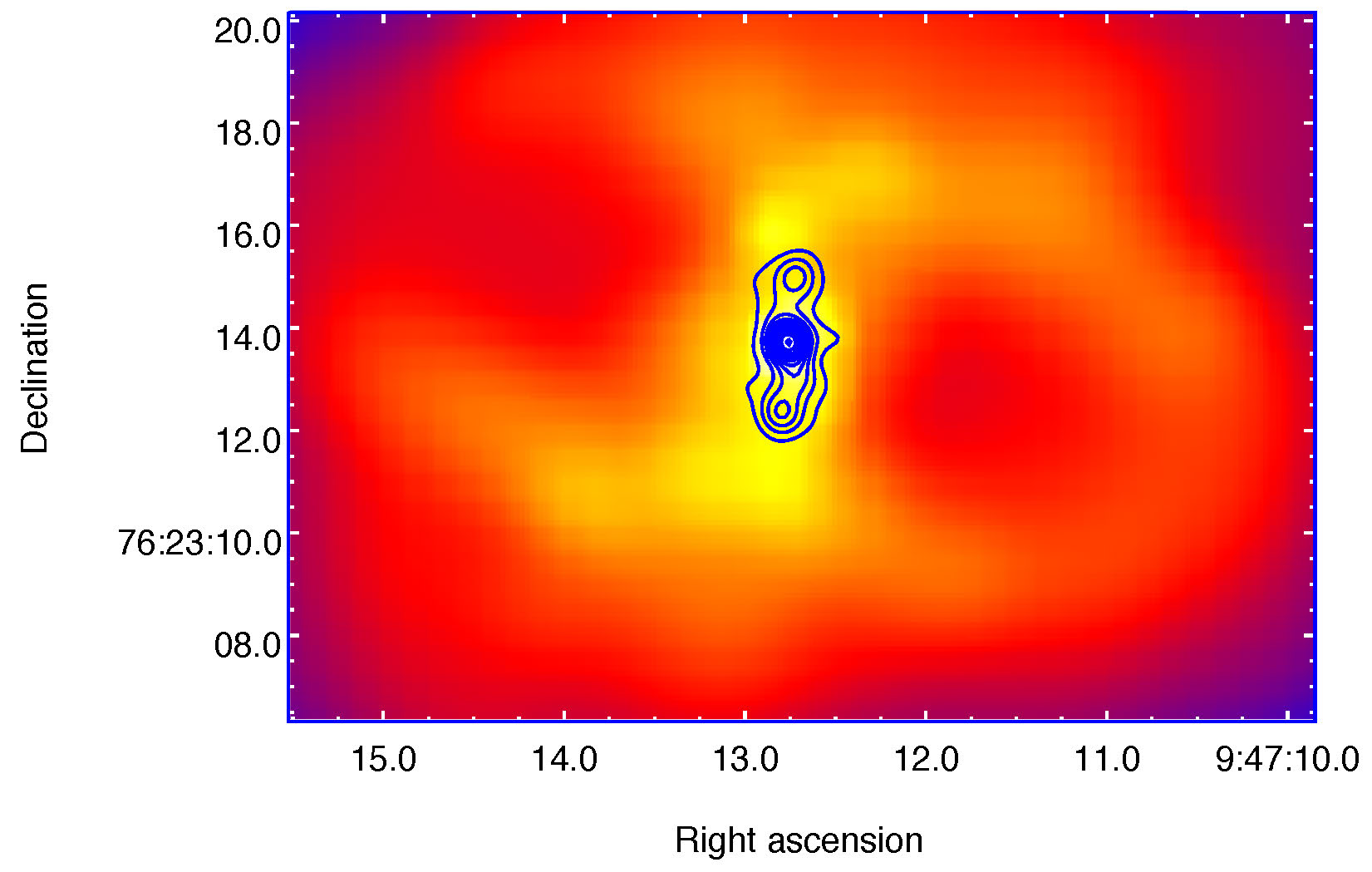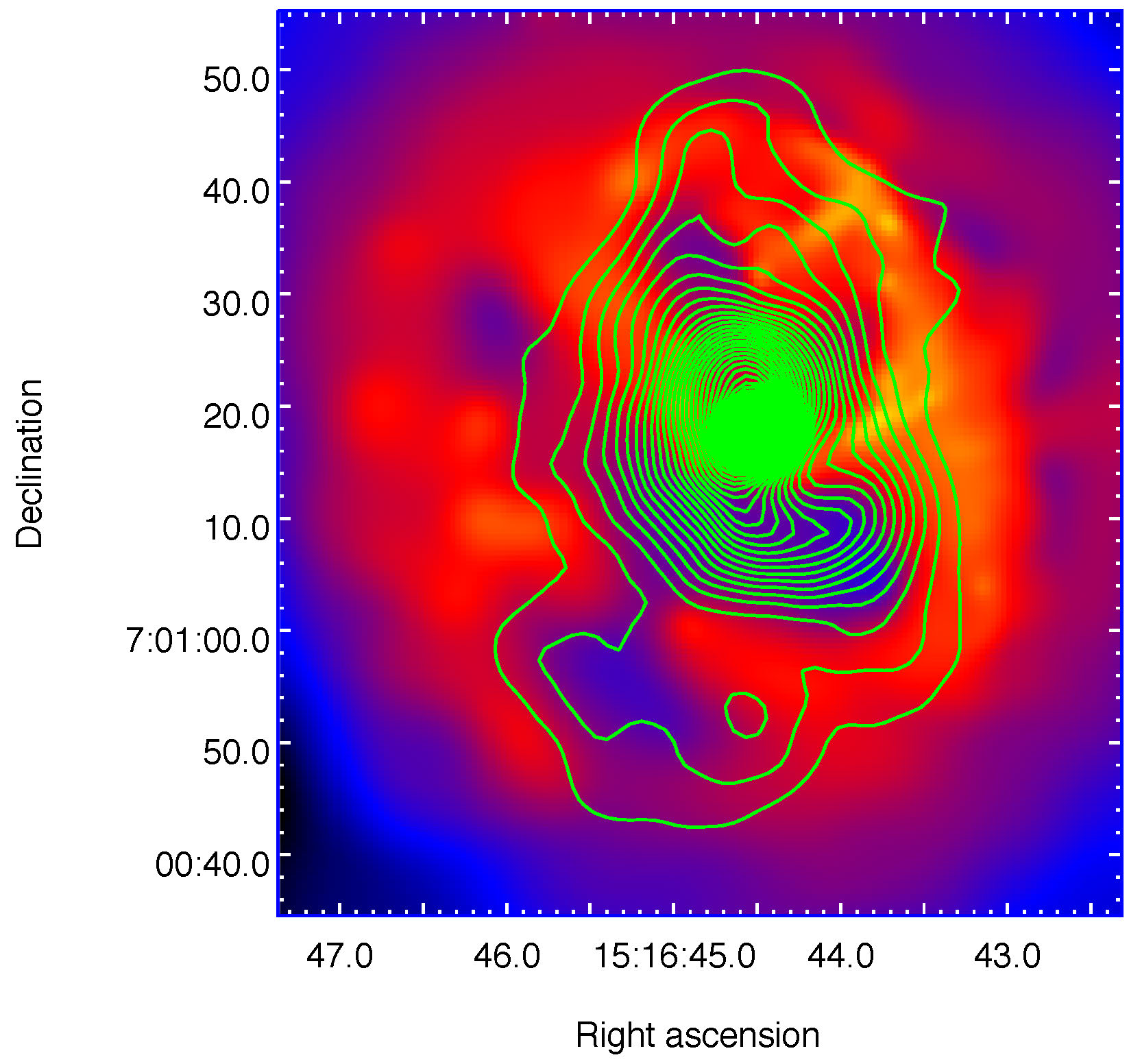Hydra A

X-ray cavities are bubbles which have been formed by the central active galactic nucleus (AGN) found at the centre of galaxy clusters, groups and giant ellipticals. There is an increasing evidence, as observed from radio/x-ray overlays, that these cavities are formed by the tip of jets of the central radio galaxy and is connected back to the nucleus, in a channel-like structure. These cavities are detected in x-ray images as surface brightness depressions. Besides, most these cavities are filled with radio-emission at 1.4 GHz while, there do exist cavities, referred to as ”ghost” bubbles which are misaligned with respect to the jet and are observed at lower radio frequency.

The formation of X-ray cavities is a consequence of AGN feedback which is responsible for the formation of other structures such as shocks and ripples. Typically, the formation of these structures may offset cooling in galaxy clusters and might presumably be one of the solutions to the classical cooling flow issue, which was proposed by Fabian (1994).RBS 797

Originally, it was believed that a cooling flow must be established at the centre of cluster of galaxy as the central atmosphere being very dense and hot, the x-ray gas must lose energy via the emission of x-ray. However, after the launching of Chandra and XMM-Newton satellites in the year 1999, it was observed that relatively little gas actually cools below 2 keV. We have investigated 9 clusters of galaxies, having redshifts $0.01 < z < 0.35$, which host x-ray cavities using CIAO 4.6 and CALDB 4.5.9. These cavities are aged about $10^7$ years. The most commonly used methods for calculating cavity ages include refill timescale $\left(t_r\right)$, buoyancy timescale $\left(t_b\right)$ and sound-crossing timescale $\left(t_s\right)$. In particular, $t_r < t_b < t_s$. The enthalpy of a cavity depends on the nature of lobes and lies between $2pV$ and $4pV$ as described by McNamara and Nulsen (2007).

The cavity power can be determined using the cavity age and enthalpy and is of the order of $10^{44}\, \textrm{ergs s}^{−1}$. In addition, the central radio source of each cluster of galaxy was studied, dealing with both strong and weak radio galaxies. Their radio luminosities at 1.4 GHz were calculated, using symbolic programming method in Matlab 2012Ra and typical value of radio luminosity $\left(\textrm{L}_{\textrm{rad}}\right)$ ranges from $0.9 − 315 \times 10^{42}\, \textrm{ergs s}^{−1}$. Using these values, together with the determined cavity power, a plot of radio luminosity against cavity power was made in the logarithmic scale, and it was found that the cavity power $\textrm{P}_{\textrm{cav}}$ varies as $$\textrm{P}_{\textrm{cav}} = \left(5 \times 10^{38\pm1}\right)\,\textrm{L}_{\textrm{rad}}^{0.13\pm0.13}$$Abell 2052

Rafferty et al. (2006) argued that the formation of x-ray cavities may be modelled by the mass accretion model. In this scenario, the jet is produced when a fraction of the gravitational binding energy of the material accreted is converted into outburst energy. While in another theory developed by Meier (1999), it is possible for cavities to be formed via the spinning of the central rotating black hole. In this model, the spin energy is converted into jet power via a torque applied by the poloidal magnetic field strength.

There could be different scenarios possible. For example, for Hydra A, the jet is aligned with the cavities while in RBS 797, the jet is roughly perpendicular to the orientation of the two cavities. On the other hand, Abell 2052 depicts an intricate ongoing activity at the core site.

On a conclusive note, the coupling between the radio galaxy and cavities still needs to be fully exploited. Jets and cavities heat the intracluster medium, thereby inhibiting cooling flow and affects accretion and galaxy growth. In fact, the nature of cavities, more precisely, its non-thermal nature opens a new world towards the study of magnetism in these characteristic sites.